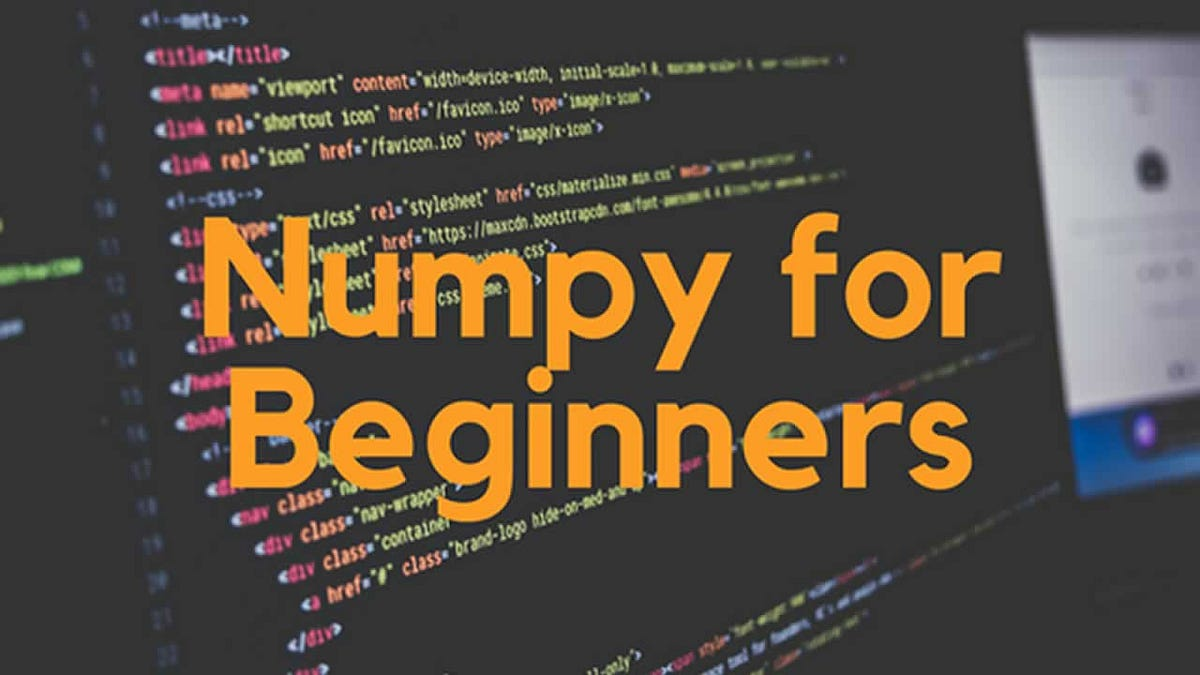#### Numpy For Beginners:Analytics Vidhya

Numpy is a library in python which is also been said as a numerical python. It is used for working with arrays, it also works in the domain of linear algebra, random numbers, and matrices. Using…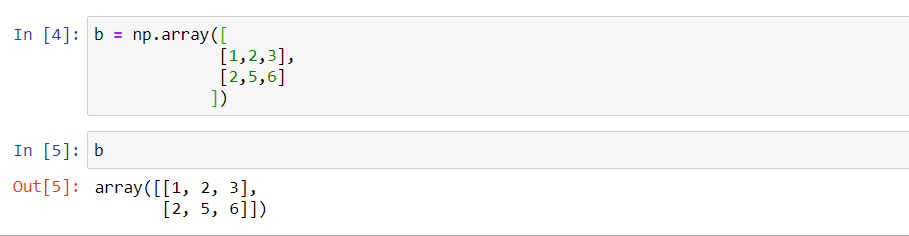#### Numpy 101Analytics Vidhya

Numpy is a extension package to Python for multi-dimensional arrays. It is designed for scientific computation. It is also Known as array oriented computing. Lets start with installation. Numpy…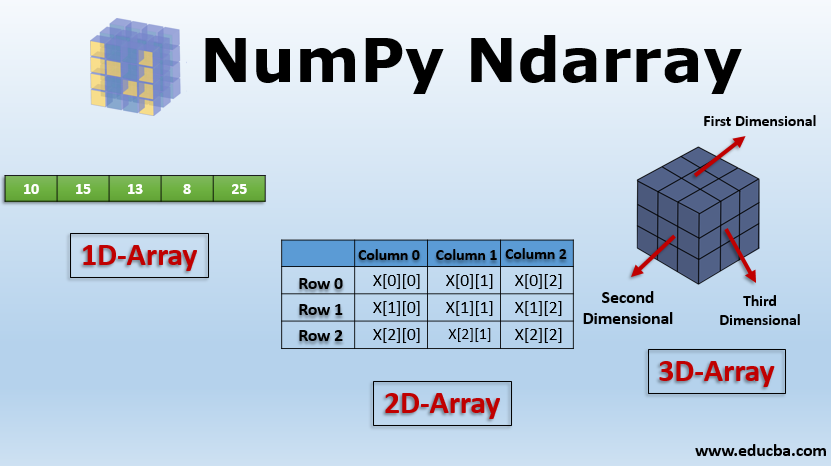#### Introduction To NumpyAnalytics Vidhya

Numpy is a general-purpose array-processing package. It provides a high-performance multidimensional array object, and tools for working with these arrays. It is the fundamental package for…#### Numpy: everything you need to knowPython in Plain English

The Numpy best introduction cheetsheet Continue reading on Python in Plain English

Read more at Python in Plain English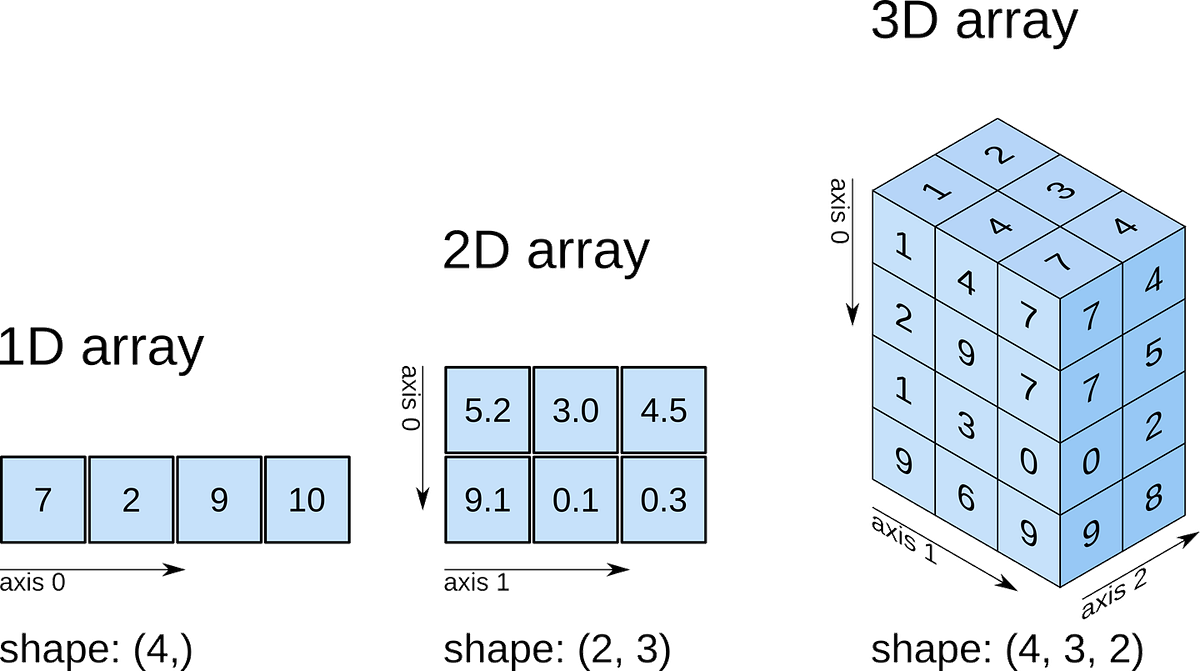#### NumPy - The very basics!Analytics Vidhya

This article is for people who have zero knowledge of NumPy so that they can get a little hang of it to kick start. NumPy is the fundamental package for scientific computing in Python.#### NumPy - The BIG picture and WORTHY insightsAnalytics Vidhya

NumPy makes it easy and pretty fast to do computation in PYTHON . NumPy is a library for the Python programming language, adding support for large, multi-dimensional arrays and matrices, along with a…...#### Introduction to Python NumpyAnalytics Vidhya

NumPy is a python library, it is written partially in python and most of the parts that require fast computation are written in C or C++. If this command fails, then use a python distribution that…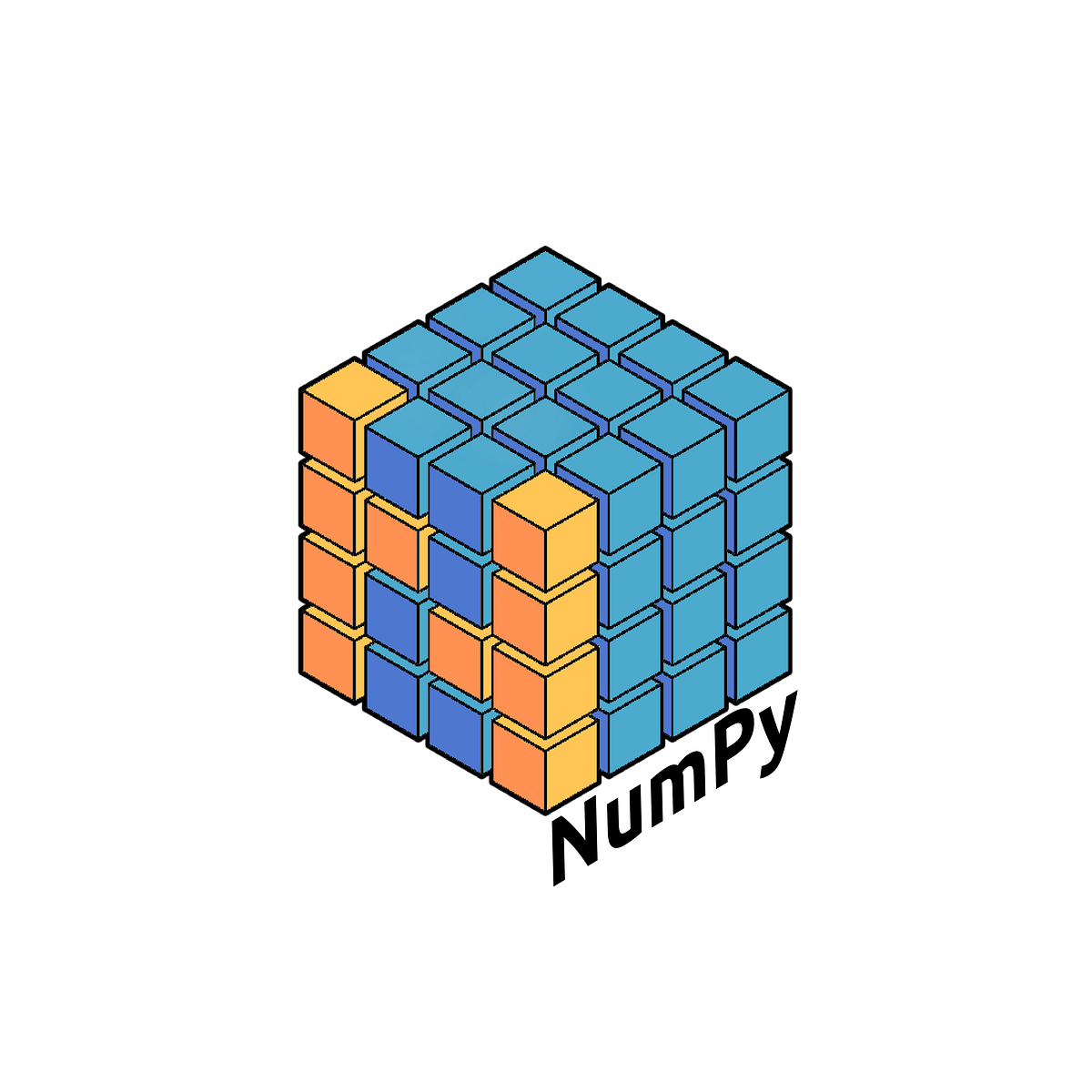#### Getting Familiar with NumpyAnalytics Vidhya

In the next few minutes, we shall get Numpy covered! An extremely popular core scientific computing Python library that every Machine Learning practitioner must be familiar with! NumPy — Numerical…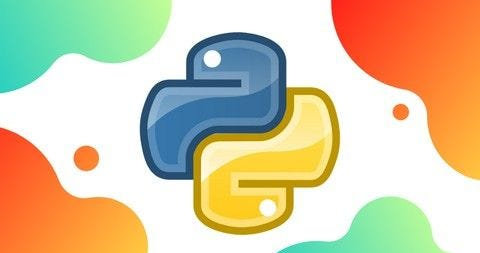#### NumPy for Data scienceAnalytics Vidhya

NumPy (Numerical Python) is an open source Python library that’s used in almost every field of science and engineering. It’s the universal standard for working with numerical data in Python, and it’s…...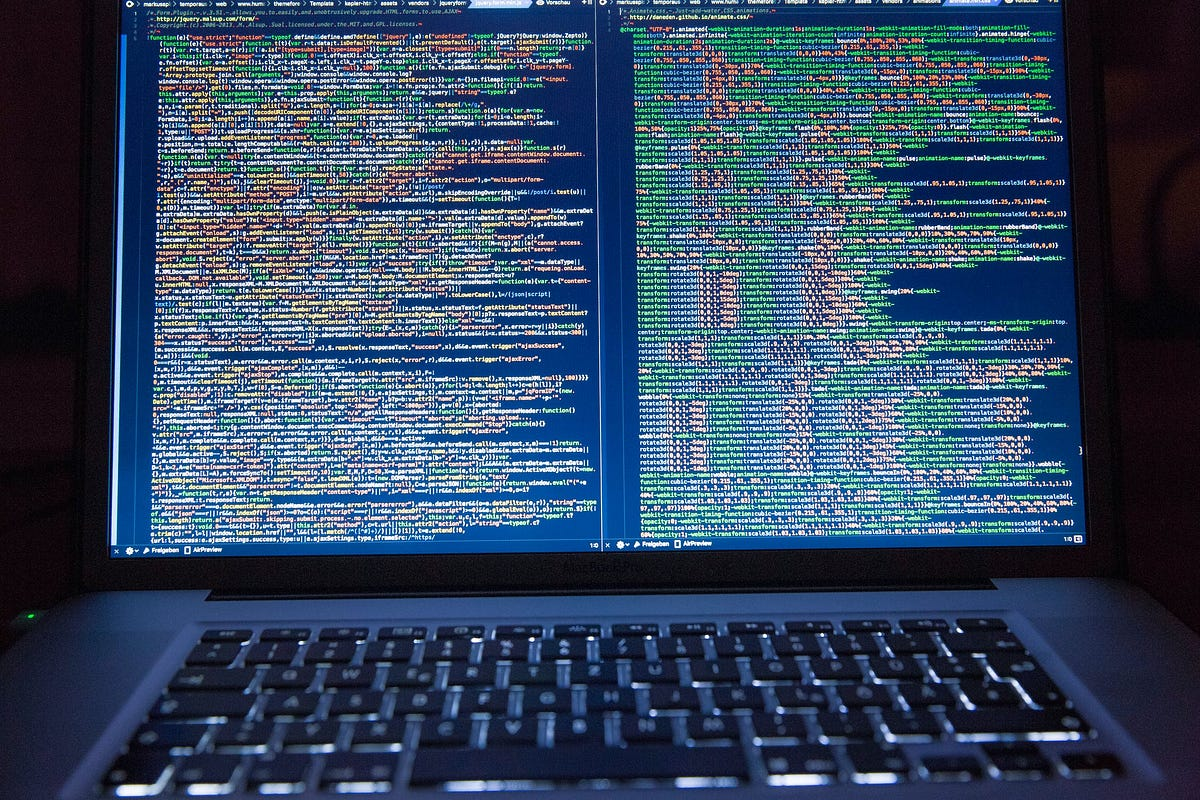#### The Glory of NumPyAnalytics Vidhya

NumPy is a library in python that provides homogeneous multidimensional arrays and matrices as well as a large collection of high-level mathematical functions. In this post, I will go over some of…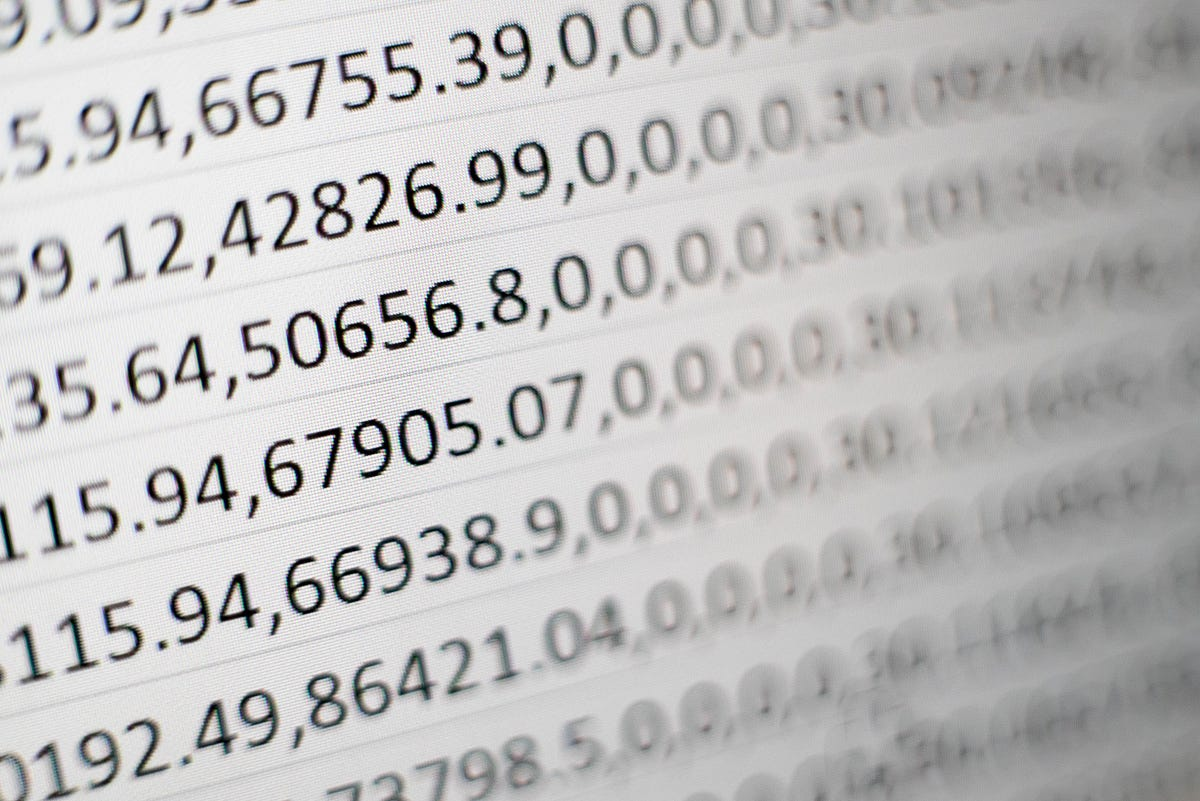#### An Easy Introduction to NumPy ArraysTowards Data Science

What, How, and Why. Continue reading on Towards Data Science

Read more at Towards Data Science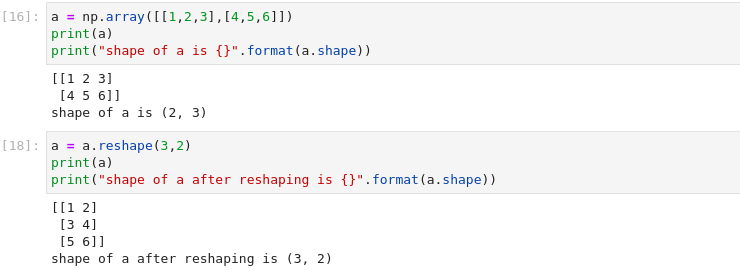#### A quick guide to getting started with NumPyAnalytics Vidhya

Whenever someone decides to enter into the world of data science, the one library he needs to learn is NumPy. NumPy Stands for Numerical Python and this article will be like a crash course in NumPy…#### NumPy ArraysLevel Up Coding

Basic operations with arrays in NumPy Continue reading on Level Up Coding

Read more at Level Up Coding#### What is NumPy?NumPy user guide

NumPy is the fundamental package for scientific computing in Python. It is a Python library that provides a multidimensional array object, various derived objects (such as masked arrays and matrices),...

Read more at NumPy user guide#### Short Introduction to NumPyTowards Data Science

Some basic Knowledge of the NumPy library and ufuncs Continue reading on Towards Data Science

Read more at Towards Data Science#### 4 NumPy Tips You Should Know!Towards Data Science

Make Working with NumPy Even Better Continue reading on Towards Data Science

Read more at Towards Data Science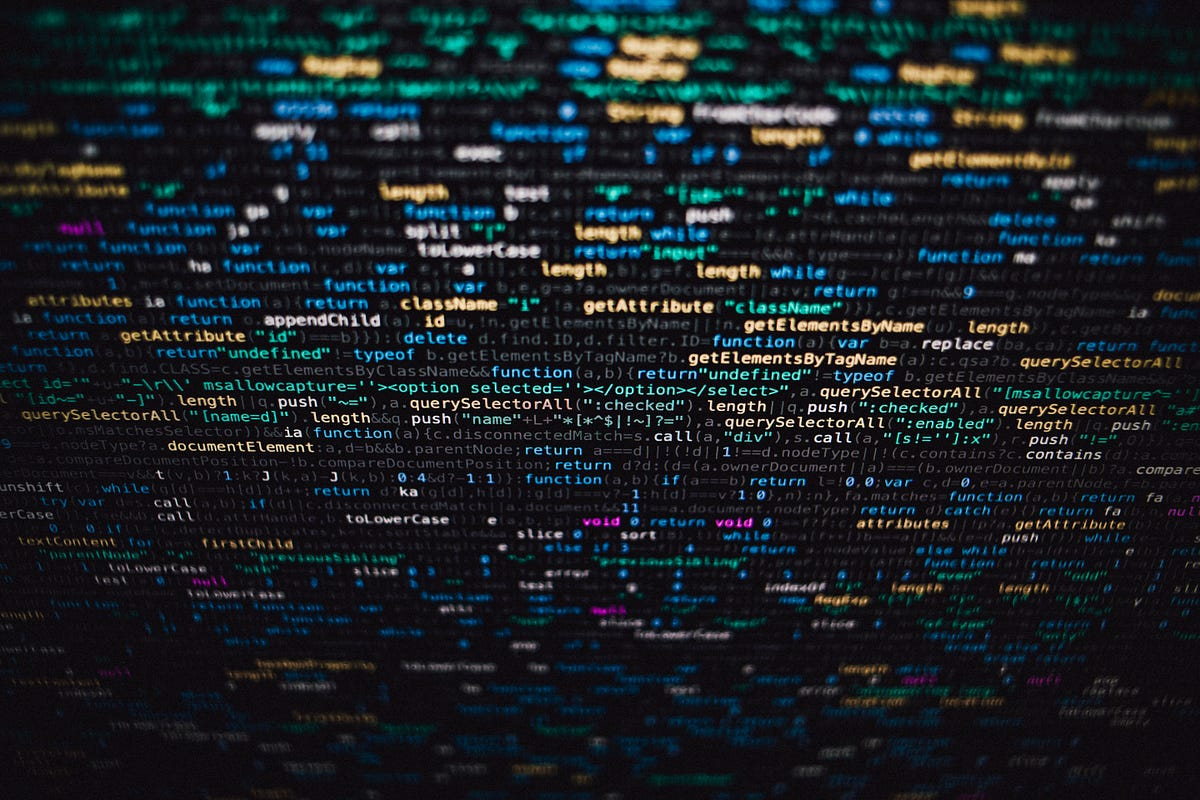#### Numpy in NumbersTowards Data Science

From Computer Science grads to Math majors, from data scientists to software engineers, from mechanical engineers to architecture students, Numpy is the quintessential Python library. Literally…

Read more at Towards Data Science

#### Computation on Numpy Arrays:Analytics Vidhya

Up until now, we have been discussing some of the basic nuts and bolts of Numpy ; in this section we will dive deep into the reasons that Numpy is so important in the Python Data Science world. The…#### Basic Introduction to NumpyAnalytics Vidhya

According to Wikipedia, NumPy is a library for the Python programming language, adding support for large, multi-dimensional arrays and matrices, along with a large collection of high-level…#### Diving into NumPyTowards Data Science

NumPy, one of the most important and basic libraries used in data science and machine learning, It consists of functionalities for multidimensional arrays, high-level mathematical functions such as…

Read more at Towards Data Science#### The Ultimate Beginner’s Guide to NumPyAnalytics Vidhya

Note: Most of the examples used to explain concepts of NumPy have been taken from Python For Data Analysis by Wes McKinney. A ndarray is a generic multidimensional container for homogeneous data…#### Getting started with NumPyAnalytics Vidhya

Getting started with NumPy. NumPy is a Python library written in C; the name NumPy comes from Numerical Python. The precompiled mathematical functions and….

#### Introduction to the NumPy LibraryPython in Plain English

An overview of the NumPy library, which is essential for scientific research and machine learning in Python. NumPy is a fundamental package for scientific computing with Python. It contains among oth...

Read more at Python in Plain English

#### What is NumPy in Python?Python in Plain English

NumPy is a popular and widely used Python library that provides powerful and efficient tools for numerical computing. NumPy stands for Numerical Python, and it is designed to enable the creation, mani...

Read more at Python in Plain English